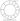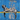# Next Larger in Circular ArrayFebruary 11, 2020

## The Problem:

A circular array is one where the next element of the last element is the first element.

You know how standard arrays look. Instead of`[1, 2, 3, 4]`, imagine the following, where after index-7, we’d move back to index-0.

Can you write a method`nextLargerNumber(nums: array)`to print the next immediate larger number for every element in the array?

Note: for any element within the circular array, the next immediate larger number could be found circularly— past the end and before it. If there is no number greater, return`-1`.

Take the following example, with an analysis for each index:

``````nextLargerNumber([3, 1, 3, 4])
// [4, 3, 4, -1]
// 3's next greater is 4
// 1's next greater is 3
// 3's next greater is 4 again
// 4 will look to the beginning, but find nothing, so -1``````

## My Solution:

So I start by creating the start array, and an empty end array. My approach is to use nested for loops to go through the array, find the next largest, and push it to the end array. I’m sure there are other ways of doing this that are more efficient but this is what I came up with (using JavaScript). Here’s the code:

``````// Solution Attempt 1

var startArray = [2, 4, 1, 2, 3, 6, 7, 2, 5, 2, 9, 6, 4];
var endArray = [];
var checksDone = false;

function nextLargeNumber() {
for (var i = 0; i < startArray.length; i++) {
for (var x = 1; x <= startArray.length; x++) {
checksDone = false;
if (x === startArray.length) {
for (var y = 0; y < i; y++) {
if (startArray[i] < startArray[y]) {
endArray.push(startArray[y]);
break;
}
}
} else if (startArray[i] < startArray[i + x]) {
endArray.push(startArray[i + x]);
break;
}
checksDone = true;
}
if (checksDone && endArray.length !== startArray.length) {
endArray.push(-1);
}
}

console.log("starting array : " + startArray);
console.log("solution array : " + endArray);
}

nextLargeNumber();``````

.

.

Seems to work! Yay for for loops! I mean, I can see why this is probably close to the worst way to do it, since we end up using a for loop, nested in a for loop, nested in a for loop (!!) to do the part of the function where it circles back to the beginning of the array if necessary. But Hey, it DOES work.

I’m going to post this and go do something else for awhile and then come back to it and see if I can get another more efficient solution figured out.

-mrWritten by Matthew Riddett who lives and works in Victoria BC, building fun and useful things. You can follow him on Twitter